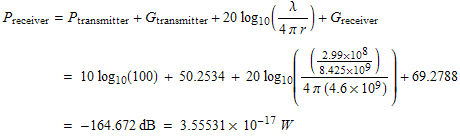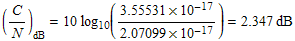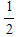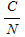"Penetrating Planets Since 2004"ECE6390 Introduction to Satellite Communications Fall, 2004
Initial ResearchIntroductionProject RequirementsNeptune FactsCalculations
Penetrating ProbesIntroductionDesignCalculationsParts & Pricing
Relay SatelliteIntroductionLink with ProbeCalculationsLink with EarthCalculationsParts & Pricing
ConclusionsSummaryTotal CostContact Info
Home » Relay Satellite » Link with Earth » Calculations
 Calculations   The associated gain of a DSN antenna is determined by, with a diameter of 34m and an efficiency η = 0.94,Similarly, the gain of the relay satellite antenna, with a diameter of 4m and an efficiency of η = 0.85, is determined to be 50.2534 dB. The power of the radio transmission will be about 100 W, the same as the probes.  Performing the link budget analysis, the signal power received at the Earth DSN dish isThe bandwidth of the signal was increased to approximately 50 kHz due to the additional redundancies of the turbo coding correction.  With a, the DSN antennas will introduce a noise ofThe Carrier to Noise ratio can then be determined byIn any digital link it is important to determine the BER.  The totalratio received at NASA's Deep Space Network was 2.347 dB.  If this signal contained no error correction, the BER would beWith such a low carrier to noise ratio, approximately 1 in every 32 bits would be lost.  However, error correction was used, which essentially yields in a BER of 0; from Figure 2 in Section Penetrating Probes » Design, the BER for a rateturbo code is extremely low for anyabove 1 dB.  A rateturbo code was used, resulting in more coding gain; therefore, an error-free communications channel can be assumed.   Next Section » References:
top of page

William W
e-mail